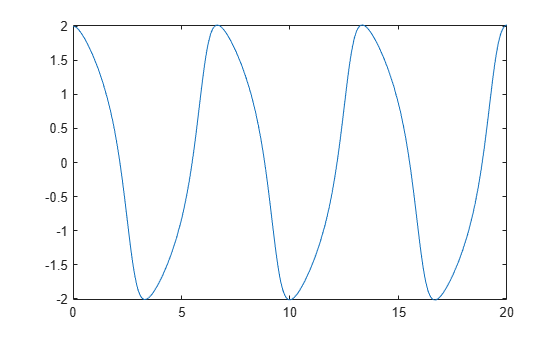# Solve a Second-Order Differential Equation Numerically

This example shows you how to convert a second-order differential equation into a system of differential equations that can be solved using the numerical solver `ode45` of MATLAB®.

A typical approach to solving higher-order ordinary differential equations is to convert them to systems of first-order differential equations, and then solve those systems. The example uses Symbolic Math Toolbox™ to convert a second-order ODE to a system of first-order ODEs. Then it uses the MATLAB solver `ode45` to solve the system.

### Rewrite the Second-Order ODE as a System of First-Order ODEs

Use `odeToVectorField` to rewrite this second-order differential equation

`$\frac{{d}^{2}y}{d{t}^{2}}=\left(1-{y}^{2}\right)\frac{dy}{dt}-y$`

using a change of variables. Let $y\left(t\right)={Y}_{1}$and $\frac{dy}{dt}={Y}_{2}$ such that differentiating both equations we obtain a system of first-order differential equations.

`$\begin{array}{c}\frac{d{Y}_{1}}{dt}={Y}_{2}\\ \frac{d{Y}_{2}}{dt}=-\left({Y}_{1}^{2}-1\right){Y}_{2}-{Y}_{1}\end{array}$`

```syms y(t) [V] = odeToVectorField(diff(y, 2) == (1 - y^2)*diff(y) - y)```
```V =  $\left(\begin{array}{c}{Y}_{2}\\ -\left({{Y}_{1}}^{2}-1\right) {Y}_{2}-{Y}_{1}\end{array}\right)$```

### Generate MATLAB Function

The MATLAB ODE solvers do not accept symbolic expressions as an input. Therefore, before you can use a MATLAB ODE solver to solve the system, you must convert that system to a MATLAB function. Generate a MATLAB function from this system of first-order differential equations using `matlabFunction` with V as an input.

`M = matlabFunction(V,'vars', {'t','Y'})`
```M = function_handle with value: @(t,Y)[Y(2);-(Y(1).^2-1.0).*Y(2)-Y(1)] ```

### Solve the System of First-Order ODEs

To solve this system, call the MATLAB `ode45` numerical solver using the generated MATLAB function as an input.

`sol = ode45(M,[0 20],[2 0]);`

### Plot the Solution

Plot the solution using `linspace` to generate 100 points in the interval [0,20] and `deval` to evaluate the solution for each point.

`fplot(@(x)deval(sol,x,1), [0, 20])`